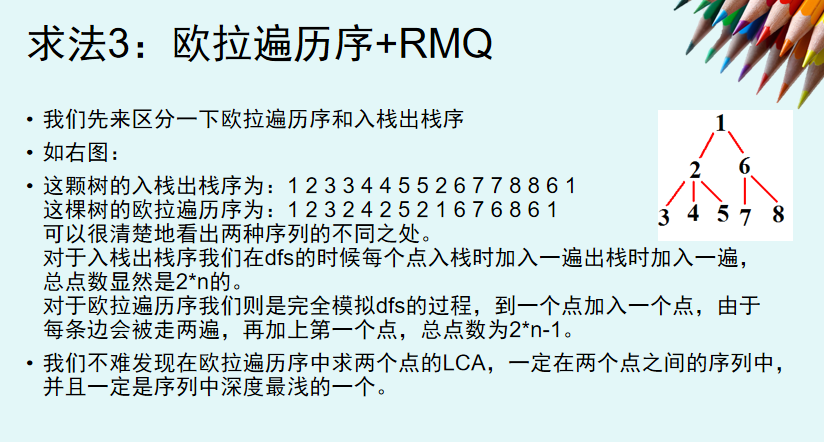# LCA 最近公共祖先

1.倍增LCA

work的时候，利用二进制拆分的思想，先把两个节点向上翻到同一个深度，再同时向上翻，直到到了lca的儿子位置，再返回f[x]（f[y]）即可。

int main()
{
for(int j=1;j<=L;j++)
for(int i=1;i<=n;i++)
{
f[i][j]=f[f[i][j-1]][j-1];
}
dep=-1;//important!!
for(int i=1;i<=n;i++)
{
int t=i;
for(int j=L;j>=0;j--)
if(f[t][j]!=0)
{
t=f[t][j];
dep[i]+=(1<<j);
}
}
}
int LCA(int x,int y)
{
if(dep[x]<dep[y]) swap(x,y);
for(int j=L;j>=0;j--)
{if(dep[f[x][j]]>=dep[y])
x=f[x][j];
}

if(x==y) return x;
for(int j=L;j>=0;j--)
if(f[x][j]!=f[y][j])
x=f[x][j],y=f[y][j];
return f[x];
}

2.树链剖分LCA

int fa[N],dep[N],son[N],top[N],size[N];//全部需要的数组
int work(int x,int y)//lca部分
{
while(top[x]!=top[y])
{
if(dep[top[x]]>dep[top[y]]) swap(x,y);
y=fa[top[y]];
}
if(dep[x]<dep[y]) swap(x,y);
return y;
}

3.Tarjan LCA

void add2(int x,int y,int numb)
{
w[++tot].nxt=pre[x];
w[tot].to=y;
w[tot].num=numb;
pre[x]=tot;
}
int fin(int x)
{
if(fa[x]==x) return x;
return fa[x]=fin(fa[x]);
}
void tarjan(int x)
{
fa[x]=x;
vis[x]=1;
for(int i=pre[x];i;i=w[i].nxt)
{
int y=w[i].to;
if(vis[y])
lca[w[i].num]=fin(y);
}
{
int y=e[i].to;
if(!vis[y])
{
tarjan(y);
fa[y]=x;
}
}
}

udpa：2019.2.1

O(1)求最近公共祖先RMQ即可O(1)查询

posted @ 2018-05-13 12:02  *Miracle*  阅读(589)  评论(1编辑  收藏  举报